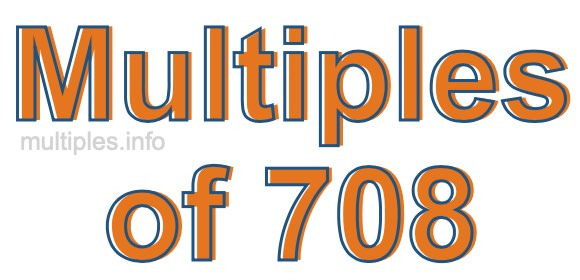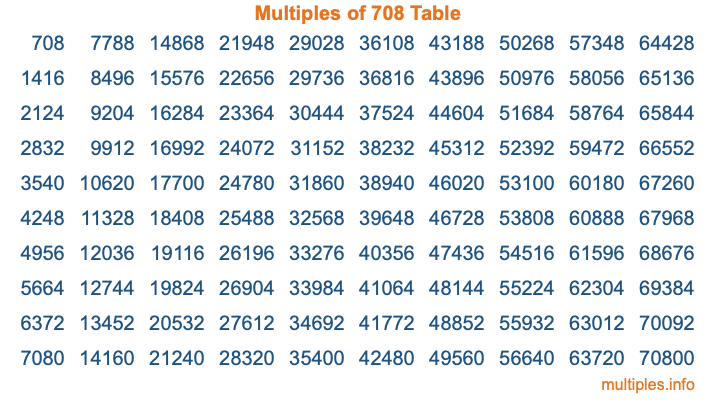Multiples of 708Welcome to the Multiples of 708 page. Here we will first teach you everything you will ever need to know about the multiples of 708, and then give you a study guide summary of everything we taught you to make sure you remember it all. Use this page to look up facts and learn information about the multiples of 708. This page will make you a multiples of seven hundred eight expert!

Definition of Multiples of 708
Multiples of 708 are all the numbers that when divided by 708 equal an integer. Each of the multiples of 708 are called a multiple. A multiple of 708 is created by multiplying 708 by an integer.

Therefore, to create a list of multiples of 708, you start with 1 multiplied by 708, then 2 multiplied by 708, then 3 multiplied by 708, and so on for as long as you want. Thus, the list of the first five multiples of 708 is 708, 1416, 2124, 2832, and 3540. To see a larger list of multiples of 708, see the printable image of Multiples of 708 further down on this page. We also have a category where you can choose any nth multiple of 708.

Multiples of 708 Checker
The Multiples of 708 Checker below checks to see if any number of your choice is a multiple of 708. In other words, it checks to see if there is any number (integer) that when multiplied by 708 will equal your number. To do that, we divide your number by 708. If the the quotient is an integer, then your number is a multiple of 708.

Is  a multiple of 708?

Least Common Multiple of 708 and ...
A Least Common Multiple (LCM) is the lowest multiple that two or more numbers have in common. This is also called the smallest common multiple or lowest common multiple and is useful to know when you are adding our subtracting fractions. Enter one or more numbers below (708 is already entered) to find the LCM.

Check out our LCM Calculator if you need more details about the Least Common Multiple or if you need the LCM for different numbers for adding and subtraction fractions.

nth Multiple of 708
As we stated above, 708 is the first multiple of 708, 1416 is the second multiple of 708, 2124 is the third multiple of 708, and so on. Enter a number below to find the nth multiple of 708.

th multiple of 708

Multiples of 708 vs Factors of 708
708 is a multiple of 708 and a factor of 708, but that is where the similarities end. All postive multiples of 708 are 708 or greater than 708. All positive factors of 708 are 708 or less than 708.

Below is the beginning list of multiples of 708 and the factors of 708 so you can compare:

Multiples of 708: 708, 1416, 2124, 2832, 3540, etc.

Factors of 708: 1, 2, 3, 4, 6, 12, 59, 118, 177, 236, 354, 708

As you can see, the multiples of 708 are all the numbers that you can divide by 708 to get a whole number. The factors of 708, on the other hand, are all the whole numbers that you can multiply by another whole number to get 708.

It's also interesting to note that if a number (x) is a factor of 708, then 708 will also be a multiple of that number (x).

Multiples of 708 vs Divisors of 708
The divisors of 708 are all the integers that 708 can be divided by evenly. Below is a list of the divisors of 708.

Divisors of 708: 1, 2, 3, 4, 6, 12, 59, 118, 177, 236, 354, 708

The interesting thing to note here is that if you take any multiple of 708 and divide it by a divisor of 708, you will see that the quotient is an integer.

Multiples of 708 Table
Below is an image of the first 100 multiples of 708 in a table. The table is in chronological order, column by column. The first column has the first ten multiples of 708, the second column has the next ten multiples of 708, and so on.The Multiples of 708 Table is also referred to as the 708 Times Table or Times Table of 708. You are welcome to print out our table for your studies.

Negative Multiples of 708
Although not often discussed or needed in math, it is worth mentioning that you can make a list of negative multiples of 708 by multiplying 708 by -1, then by -2, then by -3, and so on, to get the following list of negative multiples of 708:

-708, -1416, -2124, -2832, -3540, etc.

Multiples of 708 Summary
Below is a summary of important Multiples of 708 facts that we have discussed on this page. To retain the knowledge on this page, we recommend that you read through the summary and explain to yourself or a study partner why they hold true.

There are an infinite number of multiples of 708.

A multiple of 708 divided by 708 will equal a whole number.

708 divided by a factor of 708 equals a divisor of 708.

The nth multiple of 708 is n times 708.

The largest factor of 708 is equal to the first positive multiple of 708.

708 is a multiple of every factor of 708.

708 is a multiple of 708.

A multiple of 708 divided by a divisor of 708 equals an integer.

708 divided by a divisor of 708 equals a factor of 708.

Any integer times 708 will equal a multiple of 708.

Multiples of a Number
Here you can get the multiples of another number, all with the same attention to detail as we did for multiples of 708 on this page.

Multiples of
Multiples of 709
Did you find our page about multiples of seven hundred eight educational? Do you want more knowledge? Check out the multiples of the next number on our list!